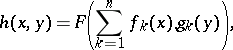# D'Alembert equation for finite sum decompositions

(diff) ← Older revision | Latest revision (diff) | Newer revision → (diff)

Consider the decomposition of a function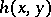into a finite sum of the form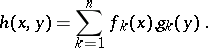For sufficiently smooth, a necessary condition for such a decomposition involves determinants of the form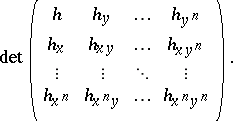These determinants were introduced in [a6] and [a7], and a correct formulation of the sufficient condition was given in [a3]; see also [a4].

A sufficient and necessary condition for not sufficiently smooth functionsdefined on arbitrary (even discrete) sets without any regularity conditions was formulated in [a3], [a4] by introducing a new, special matrix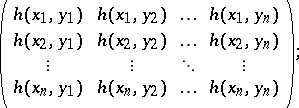Several authors have dealt with problems concerning decompositions of functions of several variables and similar questions, see, e.g., [a1], [a2], [a8]. However, several open problems in this area remain (as of 2000), e.g.: find a characterization of functionsof the form